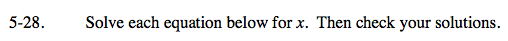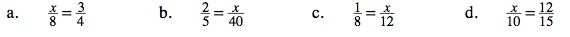### Home > CAAC > Chapter 5 > Lesson 5.1.3 > Problem5-28

5-28.Multiply both sides by 8.

$8 \cdot \frac{x}{8}=\frac{3}{4}\cdot 8$

$x=\frac{24}{4}$

x = 6

Use the same method outlined in part (a).

Use the same methodas part (a). Multiply both sides by 12.

x = 1.5

Use the methods shown in parts (a) and (c) to solve.